FreeKB - How to count records in SQL in MVC
How to count records in SQL in MVC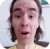First, let's count the total number of records in SQL.

``````public ActionResult Reports()
{
var xyz = db.Example.ToList();
return View(xyz);
}``````

Notice the controller points to Reports. In the Reports.cshtml file, add @Model.Count(). It is important that the word model in line 1 is all lowercase, and the word model in line 3 has a captial M.

``````@model IEnumerable<example.com.Models.Example>

@Model.Count()``````

Let's say there are a total of 429 records in SQL. In this example, www.example.com/Reports would display 429, the total number of records in SQL.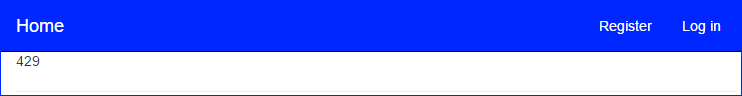Let's say you have a SQL database with a column named Location, and possible locations are:

• USA
• Europe
• Africa

Let's say we want to display the total number of records where Location equals USA. To count the number of records where Location equals USA, do the following in your Controller:

``````public ActionResult Reports()
{
var xyz = db.Example.Where(x => x.Location.Equals("USA")).ToList();
return View(xyz);
}``````

No changes are needed with the View.

``````@model IEnumerable<example.com.Models.Example>

@Model.Count()``````

In this example, www.example.com/Reports would display 274, the total number of records where Location equals USA.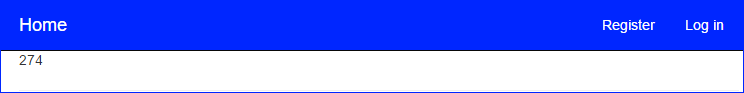Let's say you want to www.example.com/Reports to display the total number of records where Location equals USA, where Location equals Europe, and where Location equals Africa, each on a separate line.

Controller

``````public ActionResult Reports()
{
var usa = db.Example.Where(x => x.Location.Equals("USA")).Count();
ViewBag.usa= usa;

var europe = db.Example.Where(x => x.Location.Equals("Europe")).Count();
ViewBag.europe = europe;

var africa= db.Example.Where(x => x.Location.Equals("Africa")).Count();
ViewBag.africa = africa;

return View();
}``````

View

``````@ViewBag.usa
@ViewBag.europe
@ViewBag.africa``````

In this example, there are 274 records where Location equals USA, 155 where Location equals Europe, and 196 where Location equals Africa.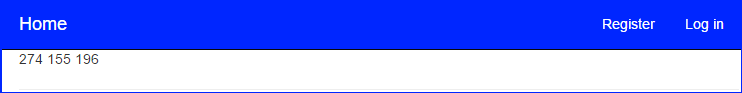Let's say you want to display the sum of USA + Europe + Africa.

View

``@(ViewBag.usa + ViewBag.europe + ViewBag.africa)``

In this example, 625 records are displayed.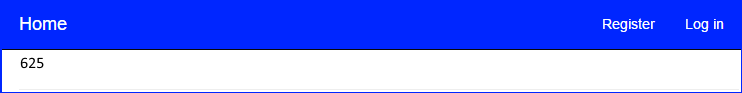We will never share your name or email with anyone. Enter your email if you would like to be notified when we respond to your comment.

Please enterin the box below so that we can be sure you are a human.